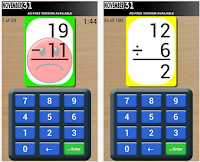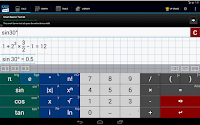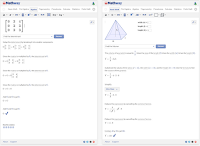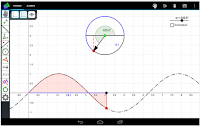# 12 Excellent Android Apps for Learning Math ~ Educational Technology and Mobile Learning

Today we curated for you this compilation of excellent Android Math apps. You can use these apps with your kids and students to improve their mathematical skills and sharpen their math mentality. The apps cover a wide variety of activities, games, exercises, and several other materials ideal for math teaching and learning. Check out the tiles below and share with us what you think of them.

1- Math Tricks“This program is designed for those who want to learn interesting mathematical tricks to speed up the calculating. These tricks will help solve part of the mathematical problems and tasks much faster than classical. Will also be helpful to those who want to hone basics such as the multiplication table.”

2- Maths Formulas Free“Available in many languages, this is a perfect app on Google Play that provides all basic formulas in mathematics. It's very convenient for all students in high school or university and engineers to look for any easy or complicated formulas.”

3- Mathematics“Calculate any formula you want and show them in a 2d or 3d plot. The natural display shows fractions, roots and exponents as you would expect it from mathematics. In a few seconds you derivate or integrate your desired function, calculate the zero points of your function und show them in the function plot. See all maxima, minima or inflection points in one view.”

4- Math Flash Cards“Children can build and improve essential math skills in: addition, subtraction, multiplication and division.Some features include: select addition & subtraction or multiplication & division together, friendly and encouraging voice, adjustable countdown timer for test-like conditions, top and bottom number ranges can be edited adjustable countdown timer for test-like conditions, top and bottom number ranges can be edited…”

5-  Complete Mathematics“Complete Mathematics App covers basic and high school maths with many options to easily learn mathematics with a maths solver. Complete Mathematics is categorized into tutorials, formulas, equations, practical application with solver, questions and solutions, maths dictionary and quiz.”

4- Mathematics DictionaryMathematics Dictionary is “equipped with quick dynamic search function (Fuzzy Logic) – The dictionary will start searching for the words while you type…”

5- Math Expert“The application "math expert" is a collection of formulas out of mathematics and physics. The special feature is that the application can calculate the formulas. The calculation is based on the motto "Tell me what you know, and I will check which calculations are possible.”

6- GMAT Math Flashcards“Master GMAT math formulas and solve GMAT questions with free flashcards for Android. Practice every day to prep for the test and get a better GMAT quant score!”

7- Graphing Calculator“Graphing Calculator by Mathlab is a scientific graphing calculator integrated with algebra and is an indispensable mathematical tool for students in elementary school to those in college or graduate school, or just anyone who needs more than what a basic calculator offers.”

9- GeoGebra“GeoGebra is free dynamic mathematics software for all levels of education that brings together geometry, algebra, spreadsheets, graphing, statistics and calculus in one easy-to-use package. Interactive teaching and learning resources created with GeoGebra can be shared and used by everyone at tube.geogebra.org”“Geometry Pad is a dynamic geometry application for Android tablets with universal appeal. Teachers can use it in a geometry class for better students engagement and deeper understanding of geometric concepts. Students would benefit from using Geometry Pad while working on geometry assignments at home as well.”

11- Mathway“Bring the solving power of Mathway to your Android device, no network access required! Get free instant answers to your math problems, or subscribe to include step-by-step work and explanations. With hundreds of millions of problems already solved, Mathway is the #1 problem solving resource available for students, parents, and teachers.”

12- FreeGeo Mathematics“Create geometric constructions and move, scale or rotate them with one or more fingers.
With the Freehandmode you can additional build your lines, circles, squares, rectangles and many more by drawing them on the screen. The system will detect it and create the object for you! For example, you can draw a freehand circle through three given points to create their circum circle.”

Source: https://www.educatorstechnology.com/2015/05/12-excellent-android-apps-for-learning-math.html